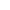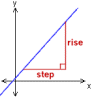Go to content
Te gradient of a straight line is found by calculating \displaystyle \frac{rise}{step}## Summary/BackgroundStraight lines have equations of the form y=mx+c, where c is the "y-intercept", in other words the point where the line crosses the y-axis. m is the gradient of the line, which can be found from the graph by constructing a triangle as shown to the right and measuring the "rise" and the "step". The gradient is given by "rise" divided by "step"You can get a better display of the maths by downloading special TeX fonts from jsMath. In the meantime, we will do the best we can with the fonts you have, but it may not be pretty and some equations may not be rendered correctly.

## Glossary

The slope of a line; the angle of its inclination to the horizontal.

### graph

A diagram showing a relationship between two variables.
The diagram shows a vertical y axis and a horizontal x axis.

### straight

The type of line produced by a linear equation.

### union

The union of two sets A and B is the set containing all the elements of A and B.

Full Glossary List

## This question appears in the following syllabi:

SyllabusModuleSectionTopicExam Year
AQA GCSE (9-1) Foundation (UK)A: GraphsA9: Plotting Straight-Line GraphsStraight-Line Graphs-
CBSE IX (India)AlgebraPolynomialsConstant, linear, quadratic and cubic-
CBSE XI (India)Coordinate GeometryStraight LinesGeneral equation of a line-
CIE IGCSE (9-1) Maths (0626 UK)5 Co-ordinate GeometryB5.4 Equations of Straight LinesStraight-Line Graphs-
Edexcel GCSE (9-1) Foundation (UK)A: GraphsA9: Plotting Straight-Line GraphsStraight-Line Graphs-
GCSE Foundation (UK)AlgebraGraphsThe straight line graph-
OCR GCSE (9-1) Foundation (UK)7: Graphs of Equations and Functions7.02a: Straight Line GraphsStraight-Line Graphs-
Universal (all site questions)GGraphsThe straight line graph-